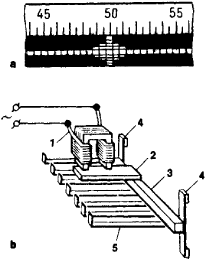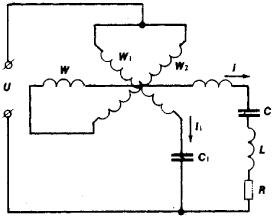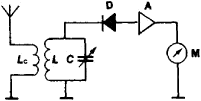# Frequency Meter

## frequency meter

[′frē·kwən·sē ‚mēd·ər]
(engineering)
An instrument for measuring the frequency of an alternating current; the scale is usually graduated in hertz, kilohertz, and megahertz.
A device cali-brated to indicate frequency of a radio wave.
McGraw-Hill Dictionary of Scientific & Technical Terms, 6E, Copyright © 2003 by The McGraw-Hill Companies, Inc.
The following article is from The Great Soviet Encyclopedia (1979). It might be outdated or ideologically biased.

## Frequency Meter

an instrument for measuring the frequency of periodic processes (oscillations). The frequency of mechanical vibrations is usually measured by means of mechanical vibration frequency meters and by electrical meters equipped with transducers to convert the mechanical vibrations into electrical oscillations.Figure 1. Electromechanical vibration frequency meter: (a) scale, registering a reading of 50 Hz, (b) diagram of the instrument; (1) electromagnet winding, (2) electromagnet armature, (3) base of the frequency meter, (4) elastic supports, (5) reeds

The simplest mechanical vibration frequency meter operates on the resonance principle and consists of a series of flexible reeds fastened at one end to a common base. The lengths and masses of the reeds are chosen in such a way that their natural vibration frequencies form a specified discrete scale, from which the value of the frequency being measured is determined. When mechanical vibrations act on the base of the meter, they cause the flexible reeds to vibrate; the highest vibration amplitude is observed on the reed whose natural vibration frequency is equal or close to the value of the frequency being measured.

The frequency of electrical oscillations is measured by means of electromechanical, electrodynamic, electronic, moving-iron, and moving-coil frequency meters. The simplest electromechanical type consists of an electromagnet and a series of flexible reeds (as in the mechanical frequency meter) on a common base that is attached to the armature of the electromagnet (Figure 1). The electrical oscillations being measured are fed to the winding of the electromagnet; the armature vibrations thereby produced are transmitted to the reeds, and the value of the frequency being measured is determined from the vibrations.

The principal element in electrodynamic frequency meters is a ratio meter with an oscillatory circuit in one of its branches that is permanently tuned to the average frequency for the measurement range of the given instrument (Figure 2). When connected to an AC circuit, the moving part of the ratio meter is deflected by an angle proportional to the phase shift between the currents in the windings of the ratio meter, which depends on the ratio of the frequency being measured to the resonance frequency of the oscillatory circuit. The measurement error of electrodynamic frequency meters ranges from 10–1 to 5 × 10–2.Figure 2. Schematic diagram of an electrodynamic frequency meter: (W) fixed coil of a ratio meter consisting of two identical parts, designed to create a uniform magnetic field; (W1) and (W2) moving coils rigidly secured together at an angle of 90°, which interact with coil W; (C), (L), and (R) electrical capacitance, inductance, and resistance, respectively, of the oscillatory circuit; (C1) capacitor to produce a phase shift of 90° between U and l1; (U) voltage whose frequency is being measured; (l) and (l1) currents in the branches of the ratio meter

The frequency of electromagnetic oscillations in the radio-frequency and microwave-frequency ranges is measured by means of electronic frequency meters (wavemeters), such as the resonant, heterodyne, and digital types.

The operation of a resonant-type frequency meter is based on the comparison of the frequency being measured with the frequency of natural oscillations in an electrical circuit (or a microwave resonator) that is tuned to resonance with the frequency being measured. The meter consists of an oscillatory circuit with a coupling loop that picks up the electromagnetic oscillations (radio waves), a detector, an amplifier, and a resonance indicator (Figure 3). During measurement, the circuit is tuned by means of a calibrated capacitor (or the plunger of a microwave resonator) to the frequency of the electromagnetic oscillations being picked up until resonance is achieved, as shown by the greatest deflection of the pointer on the indicator. The measurement error ranges from 5 × 10–3 to 5 × 10–4.

In heterodyne frequency meters, the frequency being measured is compared with a known frequency (or one of its harmonics) produced by an oscillator, or heterodyne. As the heterodyne frequency is tuned to the frequency of the oscillations being measured, beats occur at the output of a mixer (in which the frequencies are compared); after amplification the beats are indicated by the pointer on an instrument, by an earphone, or sometimes by an oscilloscope. The relative error of heterodyne frequency meters ranges from 5 × 10–4 to 5 × 10–6.Figure 3. Schematic diagram of a resonant-type frequency meter: (Lc) coupling loop, (L) and (C) oscillatory circuit (C is a calibrated variable capacitor), (D) detector (semiconductor diode), (A) amplifier, (M) indicator (microammeter or millivoltmeter)

Digital frequency meters (frequency counters) are now widely used. Their operation involves a count of the number of periods in the oscillations being measured during a specified time interval. Frequency counters consist of a device that converts the sinusoidal voltage of the frequency being measured into a train of unidirectional pulses, a gate for the pulses that opens for a certain time interval (usually from 10–4 to 10 sec), an electronic counter that registers the number of pulses at the gate output, and a digital display. Modern digital frequency counters operate over a frequency range from 10–4 to 109 hertz with a relative measurement error from 10–9 to 10–11 and a sensitivity of 10–2 volt. Such devices are used primarily for testing radio equipment and, with various measuring transducers, for measuring temperature, vibrations, pressure, strain, and other physical quantities.

Primary and secondary frequency standards, which have an error in the range from 10–12 to 5 × 10–14, function as a type of high-accuracy reference frequency meters. The rotational speed of shafts in machines and mechanisms is measured with a tachometer.

### REFERENCES

Mirskii, G. Ia. Radioelektronnye izmereniia, 3rd ed. Moscow, 1975.
Kushnir, F. V. Radiotekhnicheskie izmereniia, 3rd ed. Moscow, 1975.

E. G. BILYK

References in periodicals archive ?
Besides, L1 is the sum of the inductance of the steel strand and its series, capacitor R1 is the matching impedance, and the measuring range of the digital frequency meter module is between 20 kHz and 100 kHz.
For this purpose, a frequency meter that implements one of the Interpolated DFT method was built.
The MG frequency generation is measured by a frequency meter F, and the temperature of the MT - by an electronic thermometer T.
In conjunction, Aqua has planned improvement for Mifflin Township, including replacement of water metres and capital planning, replacement of aged and undersized mains and water service lines, replacement of fire hydrants, upgrades to the treatment facilities and installation of a new radio frequency meter reading system on all customer accounts.
The length of the impulses that reach these servos was measured by a F5041 frequency meter. The frequency meter was turned in the measurement of the duration mode with a change time of 1 s.
The outlook revision reflects CRISIL's belief that the Elymer group will report stable growth in its operating income and cash accruals, because of its newly developed radio frequency meter (RFM) technology, leading to an expected improvement in its business and financial risk profile.
This output in terms of frequency can be monitored by the frequency meter & the amplitude of the signal can be determined.
Pulse Frequency Meter. Anaesthesia 1958; 13:352-355.
The product weighs less than 4kg and features broadband power meter, frequency meter, deviation and modulation Index, SINAD and distortion measurements.
They use kit including an electromagnetic frequency meter, an infra red meter, a night vision camera and motion sensors.
"My Electromagnetic Low Frequency Meter picked up a reading of 30 milligauss, which is very high.

Site: Follow: Share:
Open / Close